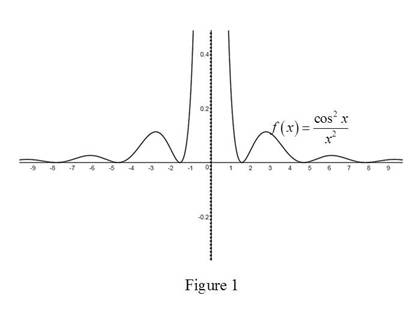# The graph of a function f ( x ) = cos 2 x x 2 and discover the asymptotes.### Single Variable Calculus: Concepts...

4th Edition
James Stewart
Publisher: Cengage Learning
ISBN: 9781337687805### Single Variable Calculus: Concepts...

4th Edition
James Stewart
Publisher: Cengage Learning
ISBN: 9781337687805

#### Solutions

Chapter 2, Problem 19RE
To determine

## To sketch: The graph of a function f(x)=cos2xx2 and discover the asymptotes.

Expert Solution

The vertical asymptote of the function f(x)=cos2xx2 is x=0.

### Explanation of Solution

Graph:

Use an online graphing calculator to draw the graph of f as shown below in Figure 1.Observation:

From the above graph, it is observed that x=0 is the vertical asymptote to the function f(x)=cos2xx2.

Recall from the definition that x=a is vertical asymptote if limxbf(x)= or limxbf(x)=.

Consider the function, f(x)=cos2xx2.

Take limit on the above expression

limx0f(x)=limx0cos2xx2=limx0(cos2x)×limx0(1x2)=(cos20)×(102)=

Thus, limx0f(x)=.

Thus, the vertical asymptote to the function f(x)=cos2xx2 is x=0.

### Have a homework question?

Subscribe to bartleby learn! Ask subject matter experts 30 homework questions each month. Plus, you’ll have access to millions of step-by-step textbook answers!